# Table Chart Questions and Answers

Table Chart Questions and Answers paper for the written examination is given below. Candidates who are looking for Data Interpretation model question paper can find in this section. Are you searching for Data Interpretation Papers? Aspirants can download Table Chart Model Papers here.

We have given free downloading links for Data Interpretation Papers. Candidates can find Data Interpretation Practice Papers on Table Chart given below. By practicing Table Chart Previous Papers, Applicants can know the difficulty level of questions.

You can find furthermore Data Interpretation Model Papers at the site given below. The Table Chart Questions and Answers Papers are updated here. Hence, interested aspirants can download the Table Chart Model Papers from the provided links.

## Data Interpretation Model Questions and Answers on Table Chart

(Q. 1-5): Study the table carefully to answer the questions that follow.

Per annum income of six men and the percentage break up of their spending pattern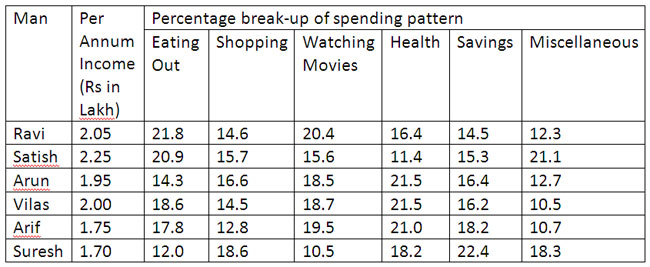1. What is the total amount of savings of all the men together?
1) Rs 1,98,460
2) Rs 1,42,524
3) Rs1 89 520
4) Rs 1,56,625
5) None of these

2. Who spends the maximum amount on shopping?
1) Ravi
2) Suresh
3) Satish
4) Arun
5) None of these

3. The amount spent by Suresh on health 1S what per cent of the total amount spent by all the men together on health? (rounded off to two digits after decimal)
1) 16.24
2) 14.60
3) 18.04
4) 12.35
5) None of these

4. The per annum income of Arif is approximately what percent of the total per annum income of all the men together?
1) 10
2) 22
3) 27
4) 19
5) 15

5. What is the ratio of the total amount spent by Ravi on eating out and watching movies together to the total amount spent by Arun on the same?
1) 156:211
2) 217:253
3) 253:217
4) 211:156
5) None of these

Directions (6 – 10): Study the following table carefully and answer the questions that follow :

Monthly Expenditure (In Thousand Rs.) By Five persons On Rent, Food, Children’s Education, Clothes And Traveling6. What is the average expenditure of person C on all the five commodities together?
(1) 6560
(2) 7660
(3) 7560
(4) 7440
(5) None of these

7. Expenditure of which person on all the five commodities together is second highest?
(1) A
(2) B
(3) C
(4) D
(5) E

8. What is the respective ratio between the expenditure of person A on food and the expenditure of person E on clothes?
(1) 16:25
(2) 17: 25
(3) 25: 17
(4) 14: 25
(5) None of these

9. Total expenditure on rent by all the persons together is what percent of expenditure of D on children‘s education?
(1) 430
(2) 425
(3) 410
(4) 416
(5) 420

10. What is difference between the expenditure of person-B on Travelling and the expenditure of person A on food?
(1) 1,000
(2) 1,200
(3) 1,500
(4) 1,600
(5) None of these

Directions (11-15): Study the table carefully to answer the questions that follow:

Number of Students Appeared and Passed In an Examination Held in Four Different Schools In Five Years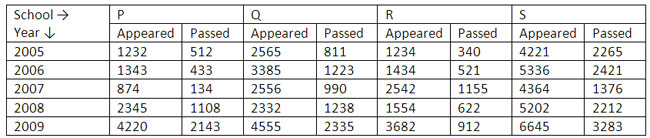11. In which year and from which school the number of students appeared in the exam were maximum?
(1) In year 2008 from School-S
(2) In year 2009 from School-S
(3) In year 2009 from School-Q
(4) In year 2008 from School – Q
(5) None of these

12. In which year was the difference between the appeared students and passed students second lowest in School R?
(1) 2005
(2) 2006
(3) 2007
(4) 2008
(5) 2009

13. What was the average number of students passed from School over all the years together ?
(1) 856
(2) 832
(3) 842
(4) 866
(5) None of these

14. Number of students passing from School-S in the year 2008 was approximately what percentage of the number of students appeared in the examination from school-Q in the year 2005?
(1) 72
(2) 78
(3) 82
(4) 76
(5) 86

15. If 25 percent of the number of students passed in the exam from school-P in the year 2005 were disqualified due to some reason, what was the number of qualified students?
(1) 442
(2) 384
(3) 432
(4) 364
(5) None of these

Q 16 – 20: Study the following table carefully and answer the questions that follow:

Number of employees from six different banks located in different cities

M=Males F=Females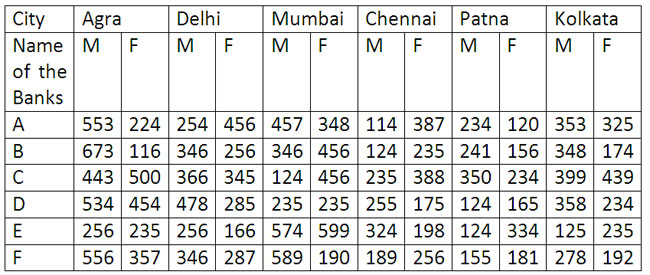16. What is the ratio of number of males to the number of female respectively in Bank D from all the cities together?
A. 496:387
B. 487:356
C. 422:385
D. 486:397
E. None of these

17. What is the ratio of the number of males to the number of females respectively in Patna from Bank A, Bank C and Bank E together?
A. 175 : 173
B. 177 : 173
C. 177 : 172
D. 175 : 172
E. None of these

18. What is the approximate average of the number of males working in all the banks together in Kolkata?
A. 350
B. B. 310
C. 340
D. 380
E. 360

19. The number of females in all the banks together in Delhi is approximately what percent of the number of males from all the banks together in the same city?
A. 88
B. 98
C. 78
D. 68
E. 58

20. The number of females in Bunk B from Agra is what percent of the females in Bank C from the same city?
A. 33.2
B. 23.2
C. 13.2
D. 28.2
E. None of these

Directions (21-25): Study the following table carefully to answer these questions.

Number of employees in different departments of five organizations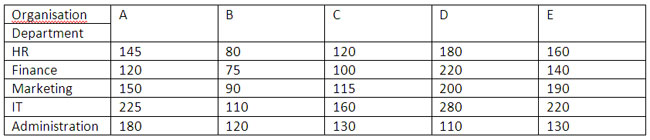21. What is the average number of employees working in Marketing department of all the organizations?
a. 149
b. 145
c. 146
d. 148
e. None of these

22. What is the total number of employees working in all the departments of organization B together?
a. 350
b. 375
c. 425
d. 475
e. None of these

23. What is the ratio between number of employees from Finance and Marketing departments together of organization B and these two departments together of organization D respectively?
a. 14:9
b. 9:14
c. 11:28
d. 28:11
e. None of these

24. What is the ratio between the total number of employees from all organizations together in HR and Administration departments respectively?
a. 132 : 137
b. 137 : 132
c. 122 : 137
d. 137 : 122
e. None of these

25. Number of employees in IT department of organization C is what per cent of the total number of employees in organization C in all the departments together?
a. 26.5
b. 25.6
c. 25.4
d. 26.4
e. None of these

Qs. 26 to 30: Study the following table carefully to answer these questions

Number of students appeared in SSC examination from five schools over the years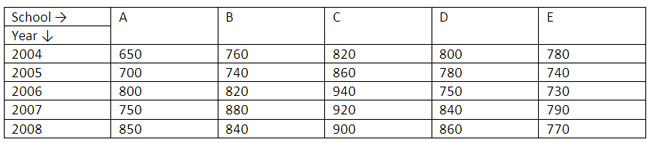26. Number of students appeared from school E in 2004 is approximately what percent of the total number of students appeared from all the schools together in that year?
A. 12
B. 28
C. 15
D. 30
E. 20

27. What is the average number of students appeared from school B for all the years?
A. 828
B. 808
C. 804
D. 812
E. None of these

28. Number of students appeared in 2006 from school A is what percent of the total number of students appeared from school A for all the years together?
A. 23(2/3)
B. 22(1/3)
C. 22(2/3)
D. 21(1/3)
E. None of these

29. What is the ratio between the total numbers of students appeared in 2004 and 2005 together from schools C and D respectively?
A. 84:79
B. 79:84
C. 48:79
D. 79:48
E. None of these

30. What is the average number of students appeared from all the schools in 2007?
A. 842
B. 856
C. 836
D. 830
E. None of these

Why Data Interpretation Table Charts?

Table chart in Data Interpretation is an important section. Various types of question are asked from Table Chart in GRE, CAT, MAT, GATE, IBPS, SBI, PO, Clerical and other examination from SSC. So, prepare well in this section.

Where can I get Data Interpretation Table Charts questions and answers with explanation?

Examyear provides you lots of fully solved Table Charts questions and answers with Explanation. Date Interpretation Solved examples with detailed answer description, explanation are given and it would be easy to understand. All aspirants can download Data Interpretation Table Charts model questions with answers as PDF files and eBooks.

Where can I get Data Interpretation Table Charts Interview Questions and Answers (objective type, multiple choices)?

Don’t search here-n-there. Here you will find Table Charts questions and answers for interview and competitive examination.

How to solve Data Interpretation Table Charts problems?

Read our explanation section. There we vividly discussed how to solve Data Interpretation Questions with examples.